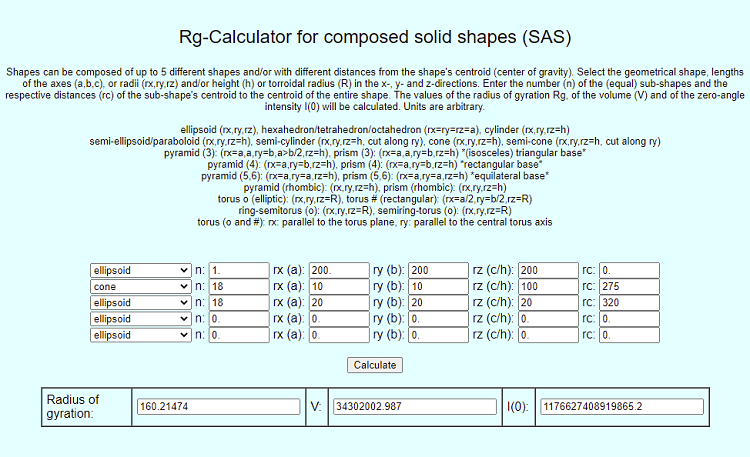Cone-spiked sphere ressembling a simplified Coronavirus shape

Select with the sliders in the upper left corner the radius of the cones (rc) and their length (rl) and the radius of the center sphere (rr), the speed of 3D-rotation of the model (speed) and the greyscale of the background (back). The apexes of the cones (18 cones distributed symmetrically) are touching the surface of the central sphere and pointing to the center of the sphere, which is also the center of gravity of the entire model. The distance from the center of gravity of the entire model to the center of gravity of the surrounding cones is equal to rr + 3/4*rl. The resulting volume (V) and the Radius of Gyration (RG) for the model with the selected parameters are displayed (assuming constant electron density) having the same (or related) units as the input parameters. A detailed nanostructural model of the Corona Virus is illustrated here. Calculating the Rg and V for the default model (rr=200, rc=25, rl=100 and with the resulting distance of the center of gravity of the 18 cones to the center of the sphere being 200 + 3/4*100 = 275) these parameters are entered as input in the Rg-Calculator for composed solid shapes as shown below.Author: M.Kriechbaum, TU-Graz (2021), e-mail: manfred.kriechbaum@tugraz.at